# Control colors in a Seaborn boxplot

Through the following examples, we cover 5 tips to customize the colors inside a boxplot figure.

## Using a color palette

Using a palette can help discriminate between several groups and get a better sens of data. You may refer to Seaborn documentation for extensive information on this topic.

``````# libraries & dataset
import seaborn as sns
import matplotlib.pyplot as plt
# set a grey background (use sns.set_theme() if seaborn version 0.11.0 or above)
sns.set(style="darkgrid")

sns.boxplot(x=df["species"], y=df["sepal_length"], palette="Blues")
plt.show()``````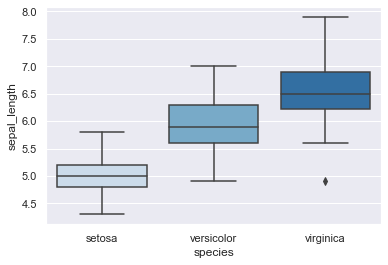## Applying a uniform color

Of course you can easily apply an uniform color to every boxes. Find a list of the numerous colors you can use here. The most common ones are
b: blue
g: green
r: red
c: cyan
m: magenta
y: yellow
k: black
w: white

``````# libraries & dataset
import seaborn as sns
import matplotlib.pyplot as plt
# set a grey background (use sns.set_theme() if seaborn version 0.11.0 or above)
sns.set(style="darkgrid")

sns.boxplot(x=df["species"], y=df["sepal_length"], color='skyblue')
plt.show()``````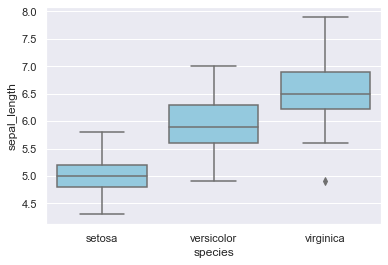## Specifying a color for each distribution

Specifying colors 'by hand' is quickly performed by creating a dictionnary composed of 'category': 'color' pairs as key:value, such as we did in the following example with my_pal.

``````# libraries & dataset
import seaborn as sns
import matplotlib.pyplot as plt
# set a grey background (use sns.set_theme() if seaborn version 0.11.0 or above)
sns.set(style="darkgrid")

my_pal = {"versicolor": "g", "setosa": "b", "virginica":"m"}
sns.boxplot(x=df["species"], y=df["sepal_length"], palette=my_pal)
plt.show()``````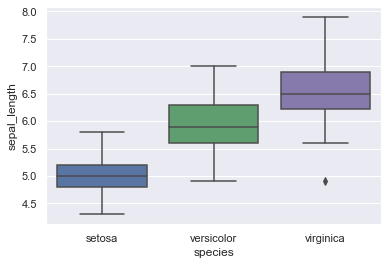## Highliting a particular group

You may want to highlight one distribution among others, and this can be done again by creating a custom palette dictionnary, such as before.

``````# libraries & dataset
import seaborn as sns
import matplotlib.pyplot as plt
# set a grey background (use sns.set_theme() if seaborn version 0.11.0 or above)
sns.set(style="darkgrid")

my_pal = {species: "r" if species == "versicolor" else "b" for species in df.species.unique()}
sns.boxplot( x=df["species"], y=df["sepal_length"], palette=my_pal)
plt.show()``````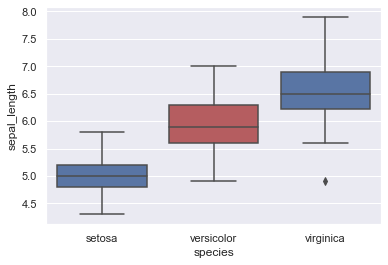I personally think that charts look better with transparency. I find out how to do it using mwaskom's Github post.
If you want to dig deeper on the matter, you can start with matplotlib documentation on Artist objects.

``````# libraries & dataset
import seaborn as sns
import matplotlib.pyplot as plt
sns.set(style="darkgrid")

# usual boxplot, plotted on a matplotlib.axes object named ax
ax = sns.boxplot(x='species', y='sepal_length', data=df)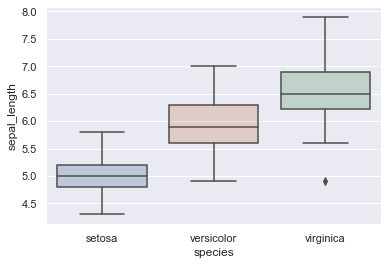👋 This document is a work by Yan Holtz. Any feedback is highly encouraged. You can fill an issue on Github, drop me a message onTwitter, or send an email pasting `yan.holtz.data` with `gmail.com`.